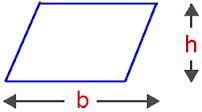# Parallelogram Formula

Geometric shape with two opposite sides and opposite angles are equal is defined as a parallelogram. This is called a parallelogram when the image is in two dimensional and if the image is a three dimensional, then it is termed as a parallelepiped. Given is the shape of a parallelogram:The formula for the area of a parallelogram is,

$\large Area=b\times h$

The formula for the perimeter of a parallelogram is,

$\large Perimeter=2\left(a + b\right)$

Where b is the base and h is the height of a parallelogram. Also, a is the side adjacent to the base.

### Solved Example

Question: Calculate the area and perimeter of a parallelogram whose base length, adjacent side and height is 5 cm, 4 cm and 11 cm respectively.

Solution:

The given parameters are:
Base length, b = 5 cm

Adjacent side, a = 4 cm
Height, h = 11 cm

The equation for area of a parallelogram is:

Area = b

$$\begin{array}{l}\times\end{array}$$
h
Area = 5
$$\begin{array}{l}\times\end{array}$$
11 = 55 cm2

Perimeter of a parallelogram is given by:
Perimeter = 2(a + b)
Perimeter = 2(4 + 5) = 2 × 19 = 18 cm